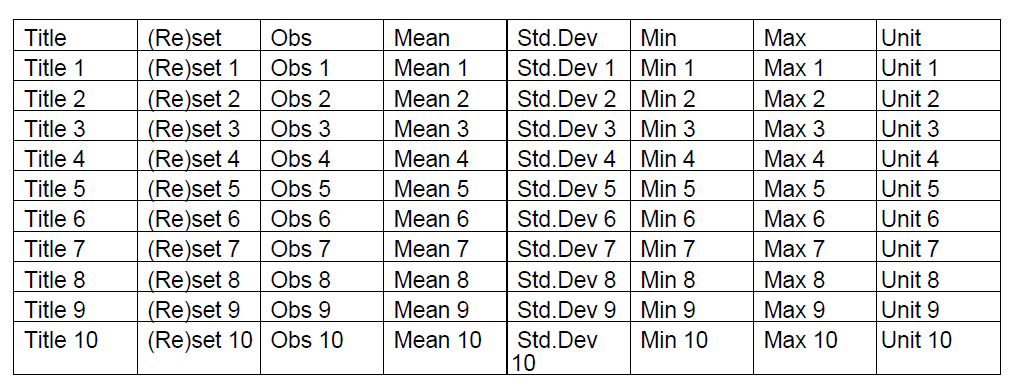I have a series of list in this list:

``private List<List<String>> tableOverallList;``

This object contains 8 values in each list. I need to place it in the table created. would like to have 2 Rows with 8 columns:

``````String[] tableTitleList = {" Title", " (Re)set", " Obs", " Mean",
" Std.Dev", " Min", " Max", "Unit"};
List<String> tabTitleList = Arrays.asList(tableTitleList);``````

Please help me to place the 1st list of values inside the List `tableOverallList` in the 2nd Row. I will try managing with the rest of the list.

``````PdfPTable table = new PdfPTable(3); // 3 columns.
PdfPCell cell1 = new PdfPCell(new Paragraph("Cell 1"));
PdfPCell cell2 = new PdfPCell(new Paragraph("Cell 2"));
PdfPCell cell3 = new PdfPCell(new Paragraph("Cell 3"));
PdfPCell cell4 = new PdfPCell(new Paragraph("Cell 4"));
PdfPCell cell5 = new PdfPCell(new Paragraph("Cell 5"));
PdfPCell cell6 = new PdfPCell(new Paragraph("Cell 6"));
PdfPCell cell7 = new PdfPCell(new Paragraph("Cell 7"));
PdfPCell cell8 = new PdfPCell(new Paragraph("Cell 8"));

Posted on StackOverflow on Jun 25, 2014 by srinivasan

That's really easy. So you have data in a nested list. For instance:

``````public List<List<String>> getData() {
List<List<String>> data = new ArrayList<>();
String[] tableTitleList = {" Title", " (Re)set", " Obs", " Mean", " Std.Dev", " Min", " Max", "Unit"};

for (int i = 0; i < 10; ) {
List<String> dataLine = new ArrayList<>();
i++;
for (int j = 0; j < tableTitleList.length; j++) {
dataLine.add(tableTitleList[j] + " " + i);
}
}

return data;
}``````

This will return a set of data where the first record is a title row and the following 10 rows contain mock-up data. It is assumed that this is data you have.

Now when you want to render this data in a table, you do this:

``````Table table = new Table(UnitValue.createPercentArray(8)).useAllAvailableWidth();

List<List<String>> dataset = getData();
for (List<String> record : dataset) {
for (String field : record) {
}
}

``````

Note that we use `Table` and `Cell` classes, because `PdfPTable` and `PdfPCell` don't exist in iText 7. The result will look like this:Resulting table

You can find the full source code here: ArrayToTable and this is the resulting PDF: array_to_table.pdf

If you only want 2 rows, a title row and a data row, change the following line:

``for (int i = 0; i < 10; ) {``

Into this:

``for (int i = 0; i < 2; ) {``

I've provided a more generic solution because it's more elegant to have generic code.

Click this link if you want to see how to answer this question in iText 5.